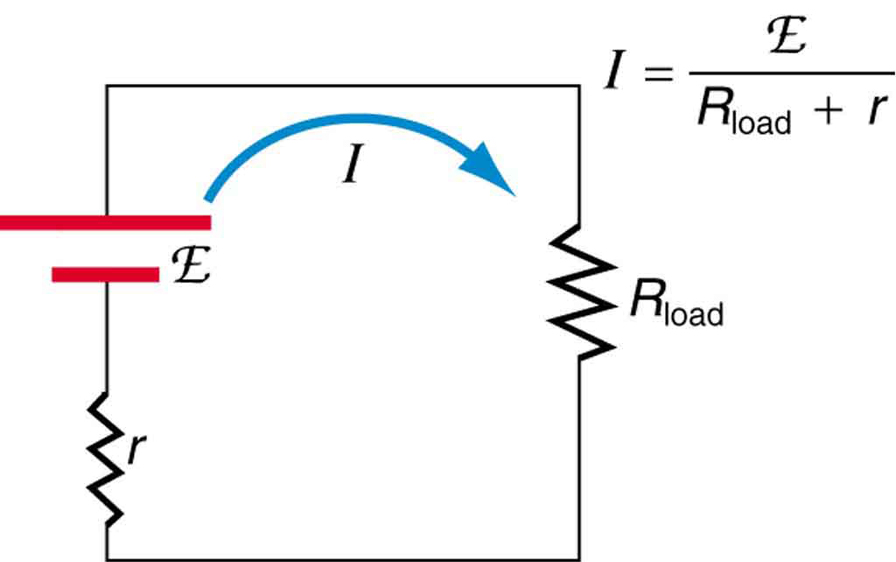# 21.2 Electromotive force: terminal voltage  (Page 3/12)

 Page 3 / 12

Why are the chemicals able to produce a unique potential difference? Quantum mechanical descriptions of molecules, which take into account the types of atoms and numbers of electrons in them, are able to predict the energy states they can have and the energies of reactions between them.

In the case of a lead-acid battery, an energy of 2 eV is given to each electron sent to the anode. Voltage is defined as the electrical potential energy divided by charge: $V=\frac{{P}_{\text{E}}}{q}$ . An electron volt is the energy given to a single electron by a voltage of 1 V. So the voltage here is 2 V, since 2 eV is given to each electron. It is the energy produced in each molecular reaction that produces the voltage. A different reaction produces a different energy and, hence, a different voltage.

## Terminal voltage

The voltage output of a device is measured across its terminals and, thus, is called its terminal voltage     $\phantom{\rule{0.25em}{0ex}}V$ . Terminal voltage is given by

$V=\text{emf}-\text{Ir},$

where $r$ is the internal resistance and $I$ is the current flowing at the time of the measurement.

$I$ is positive if current flows away from the positive terminal, as shown in [link] . You can see that the larger the current, the smaller the terminal voltage. And it is likewise true that the larger the internal resistance, the smaller the terminal voltage.

Suppose a load resistance ${R}_{\text{load}}$ is connected to a voltage source, as in [link] . Since the resistances are in series, the total resistance in the circuit is ${R}_{\text{load}}+r$ . Thus the current is given by Ohm’s law to be

$I=\frac{\text{emf}}{{R}_{\text{load}}+r}.$Schematic of a voltage source and its load R load size 12{R rSub { size 8{"load"} } } {} . Since the internal resistance r size 12{r} {} is in series with the load, it can significantly affect the terminal voltage and current delivered to the load. (Note that the script E stands for emf.)

We see from this expression that the smaller the internal resistance $r$ , the greater the current the voltage source supplies to its load ${R}_{\text{load}}$ . As batteries are depleted, $r$ increases. If $r$ becomes a significant fraction of the load resistance, then the current is significantly reduced, as the following example illustrates.

## Calculating terminal voltage, power dissipation, current, and resistance: terminal voltage and load

A certain battery has a 12.0-V emf and an internal resistance of $0\text{.}\text{100}\phantom{\rule{0.25em}{0ex}}\Omega$ . (a) Calculate its terminal voltage when connected to a $\text{10.0-}\Omega$ load. (b) What is the terminal voltage when connected to a $0\text{.}\text{500-}\Omega$ load? (c) What power does the $0\text{.}\text{500-}\Omega$ load dissipate? (d) If the internal resistance grows to $0\text{.}\text{500}\phantom{\rule{0.25em}{0ex}}\Omega$ , find the current, terminal voltage, and power dissipated by a $0\text{.}\text{500-}\Omega$ load.

Strategy

The analysis above gave an expression for current when internal resistance is taken into account. Once the current is found, the terminal voltage can be calculated using the equation $V=\text{emf}-\text{Ir}$ . Once current is found, the power dissipated by a resistor can also be found.

Solution for (a)

Entering the given values for the emf, load resistance, and internal resistance into the expression above yields

$I=\frac{\text{emf}}{{R}_{\text{load}}+r}=\frac{\text{12}\text{.}0\phantom{\rule{0.25em}{0ex}}\text{V}}{\text{10}\text{.}\text{1}\phantom{\rule{0.15em}{0ex}}\Omega }=1\text{.}\text{188}\phantom{\rule{0.25em}{0ex}}\text{A}.$

Enter the known values into the equation $V=\text{emf}-\text{Ir}$ to get the terminal voltage:

how to prove that Newton's law of universal gravitation F = GmM ______ R²
sir dose it apply to the human system
prove that the centrimental force Fc= M1V² _________ r
prove that centripetal force Fc = MV² ______ r
Kaka
how lesers can transmit information
griffts bridge derivative
below me
please explain; when a glass rod is rubbed with silk, it becomes positive and the silk becomes negative- yet both attracts dust. does dust have third types of charge that is attracted to both positive and negative
what is a conductor
Timothy
hello
Timothy
below me
why below you
Timothy
no....I said below me ...... nothing below .....ok?
dust particles contains both positive and negative charge particles
Mbutene
corona charge can verify
Stephen
when pressure increases the temperature remain what?
what is frequency
define precision briefly
CT scanners do not detect details smaller than about 0.5 mm. Is this limitation due to the wavelength of x rays? Explain.
hope this helps
what's critical angle
The Critical Angle Derivation So the critical angle is defined as the angle of incidence that provides an angle of refraction of 90-degrees. Make particular note that the critical angle is an angle of incidence value. For the water-air boundary, the critical angle is 48.6-degrees.
okay whatever
Chidalu
pls who can give the definition of relative density?
Temiloluwa
the ratio of the density of a substance to the density of a standard, usually water for a liquid or solid, and air for a gas.
Chidalu
What is momentum
mass ×velocity
Chidalu
it is the product of mass ×velocity of an object
Chidalu
how do I highlight a sentence]p? I select the sentence but get options like copy or web search but no highlight. tks. src
then you can edit your work anyway you want
Wat is the relationship between Instataneous velocity
Instantaneous velocity is defined as the rate of change of position for a time interval which is almost equal to zero
Astronomy
The potential in a region between x= 0 and x = 6.00 m lis V= a+ bx, where a = 10.0 V and b = -7.00 V/m. Determine (a) the potential atx=0, 3.00 m, and 6.00 m and (b) the magnitude and direction of the electric ficld at x =0, 3.00 m, and 6.00 m.ByByByBy OpenStaxBy OpenStaxBy Saylor FoundationBy Anonymous UserBy Stephen VoronBy OpenStaxBy Cath YuBy Jordon HumphreysBy OpenStax